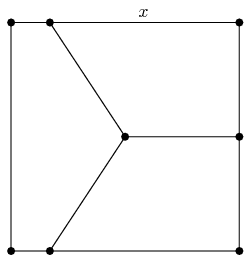# 1998 AHSME Problem 8

Geometry Level 2A square with sides of length 1 is divided into two congruent trapezoids and a pentagon, which have equal areas, by joining the center of the square with points on three of the sides, as shown. Find x, the length of the longer parallel side of each trapezoid.

×